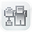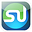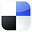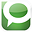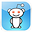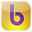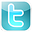Excel
STATISTICAL
Master

Combinations and Permutations

Clear and Complete - WITH LOTS OF SOLVED PROBLEMS

# Combinations and Permutations

Lots of Worked-Out, Easy-To-Understand, Graduate-Level Problems  --->
( Scroll Down and Take a Look ! )

Introduction

Combinations and Permutations are smaller groupings of objects often selected from a larger population. Objects in a Combination are selected simultaneously from the population. Objects in a Permutation are selected sequentially from the population.

## Basic Explanation of Combinations and Permutations

The concepts of combinations and permutations are closely related. A typical problem will ask how many combination or permutation groups containing x number of objects can be obtained from a larger population containing n objects.

## Difference Between Combinations and Permutations

The major difference between a combination and a permutation is when the elements of the group are chosen:

Combinations - Elements are picked simultaneously, all at once.
Permutations - Elements are picked sequentially, one after another.

If there is any order to the arrangements, it is a Permutation

******************************************************************************************

## Combination Formulas

The Number of Combinations = nPx / x! = n! / [ x! * (n - x ) ! ]
of n different objects taken x at a time Simultaneously

For example, the number of Combinations of 9 objects taken 4 at a time Simultaneously =

= nPx / x! = 9P4 / 4! = n! / [ x! * (n - x )! ]

= 9! / [ 4! * (9 - 4 )! ]

= 126

## Permutation Formulas

The Number of Permutations = nPx = n! / (n - x ) !
of n different objects taken x at a time sequentially

For example, the number of Permutations of 9 objects taken 4 at a time  Sequentially =

= nPx = 9P4 = n! / (n - x )!

= 9! / (9 - 4 )!

= 3,024

## Combination Problems:

Problem 1: Combinations of Investment Proposals

Problem: A company is evaluating 6 investment proposals. If the company selects 3 of the proposals simultaneously, how many different groups of three investment proposals can be selected?

This is a combination problem because the three investment proposals are selected simultaneously.

n = 6 = total number of investment proposals available for inclusion in each combination group

x = 3 = number of investment proposals that will be simultaneously selected to fill each combination group

The number of combinations of n = 6 different investment proposals selected x = 3 at a time simultaneously equals:

nPx / x!

= 6P3 / 3!

= n! / [ x! * (n - x )! ]

= 6! / [3! * (6 - 3)! ]

= 20

This same problem above is solved in the Excel Statistical Master with only 1 Excel formula. Everything is explained to you in SIMPLE language in the Excel Statistical Master.

Problem 2: Combinations of Newly Opened Offices

Problem: A consultancy wants to open 4 offices in 10 Northern states. Each new office will be in a different state. The offices will open all at the same time. How many different ways can these four offices be situated among the 10 possible Northern states?

This is a combination problem because the four different states
are to selected as locations simultaneously.

n = 10 = total number of states available for inclusion in each
combination group

x = 4 = number of states that will simultaneously be selected to
fill each combination group

The number of combinations of n = 10 different states available to selected at x = 4 at a time simultaneously equals:

nPx / x!

= 10P4 / 4!

= n! / [ x! * (n - x )! ]

= 10! / [ 4! * (10 - 4 )! ]

= 210

This same problem above is solved in the Excel Statistical Master with only 1 Excel formula. The Excel Statistical Master is the fastest way for you to climb the business statistics learning curve.

Problem 3: Multiple Combinations of Newly Opened Offices

Problem: A consultancy wants to open 4 offices in 10 Northern states, 3 offices in 9 Southern states, and 2 offices in 8 Eastern states. Each new office will be in a different state and all offices will be opened at the same time. How many different combinations does the company have to evaluate?

Total number of combinations =

= 10P4 / 4!  *  9P3 / 3!  *  8P2 / 2!

= 10! / [4! ( 10 - 4 )!]   *   9! / [3! ( 9 - 3 )!]   *   8! / [2! (8 - 2)!]

= 210 * 84 * 28

= 493,920

This same problem above is solved in the Excel Statistical Master with only 1 Excel formula. The Excel Statistical Master teaches you everything in step-by-step frameworks. You'll never have to memorize any complicated statisical theory.

Problem 4: Combinations of Committees

Problem: From a group of 10 men and 8 women, a committee is formed. The committee will have 3 men and 4 women. How many different ways can this committee of 3 men and 4 women be formed from the overall group of 10 men and 8 women? All committee members are picked at the same time.

This is combination problem because all committee members are picked at the same time. The problem asks how many ways can all possible combinations of 3 out of 10 men be combined with all possible combinations of 4 out of 8 women?

The Number of Combinations = Px / x! = n! / [ x! * (n - x ) ! ]

of n different objects taken x at a time Simultaneously.

Total number of combinations =

= (All possible combinations of men) * (All possible combinations of women)

= 10P3 / 3!  *   8P4 / 4!

= 10! / [3! ( 10 - 3 )!]   *   8! / [4! ( 8 - 4 )!]

= 120 * 70

= 8,400

This same problem above is solved in the Excel Statistical Master with only 1 Excel formula. The Excel Statistical Master will make you a fully functional statistician at your workplace.

Problem 5: Combinations of Sub-Groups

Problem: How many ways can a group of 12 people be divided into one group of 7 and another group of 5?

This is a combination problem because all members of any one group can be picked simultaneously.

One way to solve the problem would be to determine the total number of 7-person combinations that can be formed from 10 people and then multiply that number by the number  of 5-person combinations that can be formed from the remaining 5 people.

The Number of Combinations = nPx / x! = n! / [ x! * (n - x ) ! ]

of n different objects taken x at a time Simultaneously.

Total number of combinations =

= (total number of combination of 7 out of 12) * (total combinations of 5 out of remaining 5)

= 12P7 / 7!  *  5P5 / 5!

= 12! / [7! ( 12 - 7 )!]   *   5! / [5! ( 5 - 5 )!]

= 792 * 1

= 792

This same problem above is solved in the Excel Statistical Master with only 1 Excel formula. With the Excel Statistical Master you can do advanced business statistics without having to buy and learn expensive, complicated statistical software packages such as SyStat, MiniTab, SPSS, or SAS.

## Permutation Problems:

Problem 6: Permutations of Delivery Routes

Problem: A milkman makes 7 deliveries on his route. How many different sequences can he make to complete all 7 stops?

This is a permutation problem because the stops are done sequentially.

n = 7 = total number of objects initially available for inclusion in each permutation group

x = 7 = number of objects that will sequentially fill the permutation group

The number of permutations of n = 7 different stops taken x = 7 at a time sequentially equals:

nPx

= 7P7

= n! / (n - x )!

= 7! / (7 - 7)!

= 5,040

This same problem above is solved in the Excel Statistical Master with only 1 Excel formula. You'll finally have a solid understanding of business statistics with the Excel Statistical Master.

Problem 7: Permutations of Seating Arrangements

Problem: How many ways can 5 people be seated on a sofa if only 3 seats are available and the 3 seats are filled sequentially by the available 5 people?

This is a permutation problem because the elements of the permutation group are filled sequentially.

n = 5 = total number of objects initially available for inclusion in each permutation group

x = 3 = number of objects that will sequentially fill the permutation group

The number of permutations of n = 5 different people seated x = 3 at a time sequentially equal:

nPx

= 5P3

= n! / (n - x )!

= 5! / (5 - 3)!

= 60

This same problem above is solved in the Excel Statistical Master with only 1 Excel formula. You'll be able to grasp your statistics course a LOT easier with the Excel Statistical Master.

Problem 8: Permutations of Executive Groups

Problem: A group of 9 people needs to appoint 1 person to be group president, another person to be group vice president, and a third person to be group treasurer. If the group first votes for the president, then votes for the vice president, and finally votes for the treasurer, how many different executive groups can be created from the original 9 people?

This is a permutation problem because the elements of the permutation group are filled up sequentially.

n = 9 = total number of objects initially available for inclusion in each permutation group

x = 3 = number of object that will sequentially fill the permutation group

The number of permutations of n = 9 different people elected x = 3 at a time sequentially equals:

nPx

= 9P3

= 9! / (9 - 3 )!

= 504

This same problem above is solved in the Excel Statistical Master with only 1 Excel formula. The Excel Statistical Master is for you if you want to know how to apply statistics to solve real world business problems.

Problem 9: Permutations of Book Arrangements

Problem: How many ways can 3 books be placed next to each other on a shelf one at a time?

This is a permutation problem because the elements of the permutation group are filled sequentially.

n = 3 = total number of initially objects available for inclusion in each permutation group

x = 3 = number of object that will sequentially fill the permutation group

The number of permutations of n = 3 different books placed x = 3 at a time sequentially equals:

nPx

= 3P3

= n! / (n - x )!

= 3! / (3 - 3)!

= 6

This same problem above is solved in the Excel Statistical Master with only 1 Excel formula. If you found your statistics book confusing, You'll really like the Excel Statistical Master. Everything is explained in simple, step-by-step frameworks.

Problem 10: Permutations of Letter Groups

Problem: From the following six letters: A, B, C, D, E, F, how many groups of 3 letters can be created if none of the letters from the original 6 are repeated in any group?

This is a permutation problem because none of the letters can be repeated. When the first letter of one of the permutation groups is chosen, there are only five remaining letters to choose from. Thus, the elements of the permutation group are filled sequentially.

n = 6 = total number of objects initially available for inclusion in each permutation group

x = 3 = number of objects that will sequentially fill the permutation group

The number of permutations of n = 6 different letters placed x = 3 at a time  sequentially equally:

nPx

= 6P3

= n! / (n - x )!

= 6! / (6 - 3)!

= 120

This same problem above is solved in the Excel Statistical Master with only 1 Excel formula. You'll be able to grasp your statistics course a LOT easier with the Excel Statistical Master.

If You Like This, Then Share It...